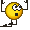# What is the difference between the resistance force and the friction coefficient?

• I
tymi9
Hi
I have been wondering what is the difference between resistance force and friction coefficient. While doing research online I could not find a clear answer. Maybe someone here will nowMentor
Well, the units for one. The friction force has units of Newtons, and the friction. Coefficient is dimensionless.

•Homework Helper
2022 Award
I will assume that "resistance force" is another name for the more common "frictional force".
When two surfaces are ~smooth it is approximately true that the friction force is proportional to how strongly the surfaces are pushed together (this is the Normal force). And so we write that $$F_{friction}=\mu N$$

Homework Helper
Gold Member
Hi
I have been wondering what is the difference between resistance force and friction coefficient. While doing research online I could not find a clear answer. Maybe someone here will nowOne is a force, the other is a ratio between two forces: normal and friction.

tymi9
Oh ok now I get it. thanks

However, is there a way to calculate the friction coefficient?

Homework Helper
2022 Award
Not in a simple way. You should well know that it depends upon a lot of different things (like cleanliness of surfaces, moisture, temperature, etc). I think you can look up measured values for various combinations of surfaces. And the supposition that the ratio is constant is does not always hold. It is overemphasized in introductory physics because the problems are easy to formulate !

•jbriggs444, PeroK and bob012345
Homework Helper
Gold Member
••tymi9 and bob012345
Mentor
Oh ok now I get it. thanks

However, is there a way to calculate the friction coefficient?
It is not simple to calculate, but it is rather straightforward to measure.

•hutchphd and bob012345
Arjan82
Let's just put here that resistance of an object can consist of multiple effects, friction is just one:
- Frictional resistance
- Form or pressure resistance (for not so aerodynamically shaped objects also called bluff body resistance)
- Wave resistance (only applicable to floating objects like ships)

So if you say what is the difference between a resistance force and frictional resistance force (I'm assuming both as a force now), then the resistance force usually contains frictional resistance but may contain other types of resistance as well, and is therefore always at least as big as the frictional resistance.